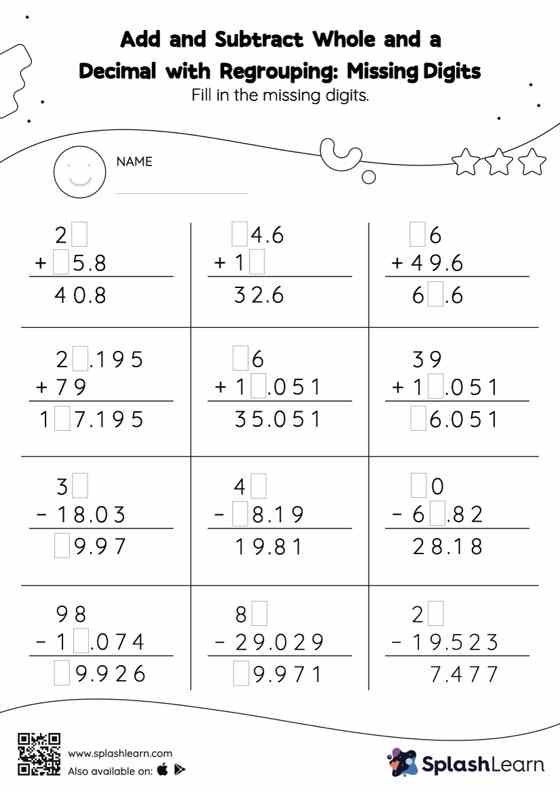# Add and Subtract Whole and a Decimal with Regrouping: Missing Digits Worksheet

Home > Add and Subtract Whole and a Decimal with Regrouping: Missing DigitsWhile adding and subtracting decimals, students use the relationship between addition and subtraction to find the missing number. They also need to regroup numbers in add and subtract whole and a decimal with regrouping worksheet utilizing the relationship between ones, tenth, hundredth, thousandth, etc., To get to the result. In this worksheet, numbers are laid one on top of another (vertical format). This encourages students to use the inherent place value structure to solve the problems.